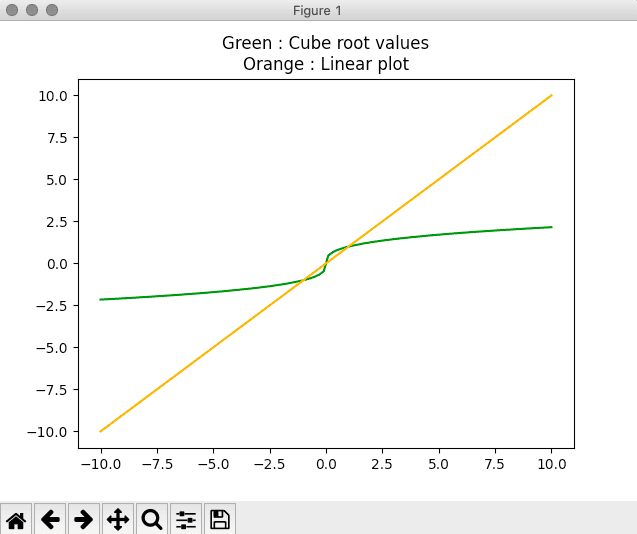# np.cbrt: How to Calculate Cube Root in Numpy

To find a cube root in numpy, use the np.cbrt() method. The numpy cbrt() function returns the cube root of every array element.

## np.cbrt

The np.cbrt() is a numpy library function that finds a cube root of every element in a given array. The Numpy cbrt() method accepts four parameters and returns the cube root of each value in the input array.

### Syntax

`numpy.cbrt(arr1, out=None, where=True, dtype=None)`

### Parameters

arr1: Input array_like containing the elements whose cube root we need to find.

out : (ndarray, None, or tuple of ndarray) [Optional parameter] It defines the alternate array in which the resulting output product is to be placed. This must have the same or broadcastable shape as the expected output.

where : (array_like) [Optional parameter] These are the items to be included.

dtype : [Optional parameter] It defines the type of the returned array.

### Return Value

The cube root of each element in the passed array is in the form of an array.

See the following figure.#### Note:

1. The cube root of complex numbers cannot be calculated using this method.
2. The cbrt() method returns the empty array if an input array is blank.
3. The cbrt() method can show a parabolic plot of the cube root function.

### Examples

The following example demonstrates the use of the numpy cbrt() method.

```import numpy as np

arr1 = [1, 8, 27, 64]
arr2 = np.cbrt(arr1)
print(arr2)
```

#### Output

`[1. 2. 3. 4.]`

In this example, we first imported numpy, defined an array of cube elements, and then used the np.cbrt() method to find the cube root of each element.

## Checking for complex numbers

The following code example demonstrates the case where an array element is a complex number.

```import numpy as np

arr1 = [3+4j]
arr2 = np.cbrt(arr1)
print(arr2)
```

#### Output

```Traceback (most recent call last):
File "app.py", line 4, in <module>
arr2 = np.cbrt(arr1)
TypeError: ufunc 'cbrt' not supported for the input types, and the inputs could not be safely coerced to any supported types according to the casting rule ''safe''```

The interpreter throws the TypeError: ufunc ‘cbrt’ not supported for the input types, and the inputs could not safely coerced to any supported types.

## Find the parabolic plot of the cube root function.

The following code example demonstrates the parabolic plot of the cube root function.

```import numpy as np
import matplotlib.pyplot as plt

x = np.linspace(start=-10, stop=10, num=100, endpoint=True)

plt.title("Green : Cube root values\nOrange : Linear plot")
plt.plot(x, np.cbrt(x), color='green')

plt.plot(x, x, color='orange')
plt.show()```

#### Output## Passing an empty array

The following code example demonstrates the case where an empty array is passed.

```import numpy as np

arr1 = []
arr2 = np.cbrt(arr1)
print(arr2)
```

#### Output

`[]`

It will return an empty array.

## Passing where parameter

The following code example shows the use of the where parameter to specify which elements to find the cube root of. For the remaining elements, the garbage value gets returned.

See the following code.

```import numpy as np

arr1 = [1, 8, 27, 64, 125]
arr2 = np.cbrt(arr1, where=[True, False, True, False, True])
print(arr2)
```

#### Output

```[1.00000000e+00 2.31584178e+77 3.00000000e+00            nan
5.00000000e+00]```

In this example, we are passing a where parameter which takes True or False values.

## Specifying dtype

The following example demonstrates the case where dtype is to specify the data type of the elements.

```import numpy as np

arr1 = [1, 8, 27]
arr2 = np.cbrt(arr1, dtype=np.double)
print(arr2.dtype == np.int)

arr1 = np.array([1, 8, 27], dtype=np.double)
print(np.cbrt(arr1).dtype == np.double)
print(np.cbrt(arr1).dtype == np.int)
```

#### Output

```False
True
False```

The following example demonstrates the application of this method in a simple programming context.

```import numpy as np

n = int(input("Count: "))
numbers = []

for i in range(n):
numbers.append(int(input()))

numbers = np.cbrt(numbers)
res = sum(numbers)

print(res)
```

#### Output

```Test Case 1:
->python3 example7.py
Count: 5
1
8
27
64
125
15.0

Test Case 2:
->python3 example7.py
Count: 3
1
8
27
6.0
```

## Conclusion

Numpy cbrt() function returns the cube-root of an array, element-wise.

In this example, we have seen how the np cbrt() function works and pass the different arguments to illustrate the different outputs.